# Chapter 3: Objects

## Chapter Goals

• To become familiar with objects
• To learn about the properties of several sample classes that were designed for this book
• To be able to construct objects and supply initial values
• To understand member functions and the dot notation
• To be able to modify and query the state of an object through member functions
• To write simple graphics programs containing points, lines, circles and text
• To be able to select appropriate coordinate systems
• To learn how to process user input and mouse clicks in graphic programs
• To develop test cases that validate the correctness of your program

## Constructing Objects

• An object is a value that can be created, stored and manipulated in a programming language.
`	string greeting = "Hello";`
• Every object in C++ must belong to a class.
• A class is a data type (like int or double) that is programmer defined, but int and double are defined by the designers of the C++ language.
• The text contains a Time, Employee, and 4 shape classes for us to learn to work with classes.
• Remember, these are programmer defined and not part of standard C++.

## Constructing Objects (Time class)

• To use the Time class we must include the file that contains its definition:
`#include "ccc_time.h"`
• We use " " instead of < > because the file is not a standard header.
• The name stands for Computing Concepts with C++ Essentials.
• Creating any object is called construction.
• We can construct an object just like we create any variable.
`Time sometime; `
• We initialize an object by passing construction parameters when the object is created.
`Time day_end = Time(23, 59, 59); /* the last second of the day */`
The shorthand in this very common situation is:
`Time day_end(23, 59, 59); /* the last second of the day */`
• Creating objects without parameters is called default construction. No parameters means not parenthesis! The Time class creates an object with the current time.
`Time now; /* the time this object is created */Time later(); /* NO! */`
• An object can be created anytime by supplying the class name with (or without) parameters. The assignment operator changes the value of the variable-object.
`sometime = Time(12, 5, 18); /* we already declared sometime */`

## Constructing Constant Objects (Syntax 3.1)

Syntax 3.1 : Constant Object Construction

`Class_name(construction parameters);`
 Example: `Time(19, 0, 0);` Purpose: Construct a new object for use in an expression.

## Constructing Objects (Syntax 3.2)

Syntax 3.2 : Object Variable Definition

`Class_name variable_name(construction parameters);`
 Example: `Time homework_due(19, 0, 0);` Purpose: Define a new object variable and supply parameter values for initialization.

## Using Objects

• A function applied to an object with the dot notation is called a member function.
• The Time class has several member functions used to find the state of the object:
`now.get_seconds(); /* returns the seconds value of now */now.get_minutes(); /* returns the minutes value of now */now.get_hours(); /* returns the hours value of now */`

## Using Objects

• The Time class does not have any member functions used to set the state of the object to avoid abuse:
`now.set_hours(2); /* NO! Not a supported member function */now.set_hours(9999); /* NO! Doesn't make sense */`
• The Time class does provide other member functions to facilitate other actions:
`now.add_seconds(1000); /* Changes now to move by 1000 seconds */now.seconds_from(day_end); /* Computes number of seconds between now and day_end */`

## Real-Life Objects

• Object-oriented programming allows us to easily model entities from real life.
• As an example, the text provides a simple Employee class:
• All employees have names and salaries which are set at construction.
`Employee harry("Hacker, Harry", 45000.00);`
• Both attributes can be queried.
`cout << "Name: " << harry.get_name() << "\n";cout << "Salary: " << harry.get_salary() << "\n";`
• Only salary can be set.
`harry.set_salary(new_salary);`

## Displaying Graphical Shapes

• Console applications read input from the keyboard (using cin) and display text output on the screen (using cout).
• Graphics applications read keystrokes and mouse clicks and display graphical shapes such as lines and circles through a window object called cwin.
`Circle c;...cout << c; /* NO! Won't display the circle */cwin << c; /* The circle will appear in the graphics window */`
• To use graphics program you must include the header ccc_win.h.
• This graphics package was created for use in the textbook (it's not part of standard C++).

## Graphics Structures (Points)

• A point has an x- and y-coordinate.
`cwin << Point(1 ,3);`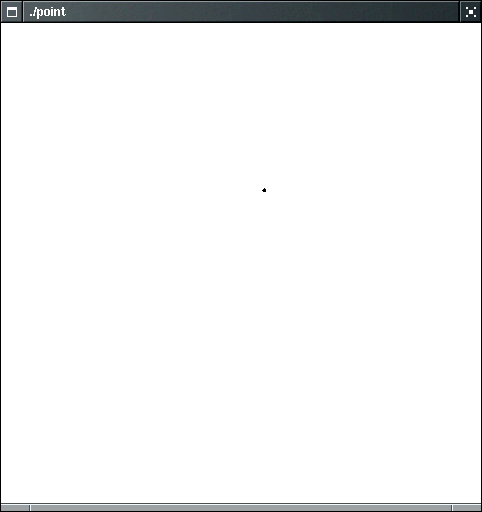## Graphics Structures (Circles)

• A circle is defined by a center point and a radius.
`Point p(1, 3);cwin << p << Circle(p, 2.5);`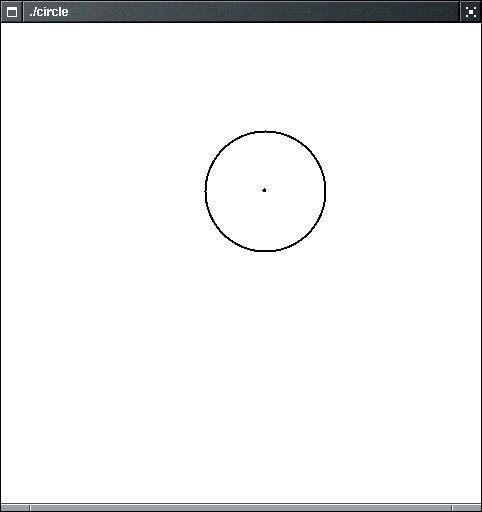## Graphics Structures (Lines)

• Two points can be joined by a line.
`Point p(1, 3);Point q(4, 7);Line s(p, q);cwin << s;`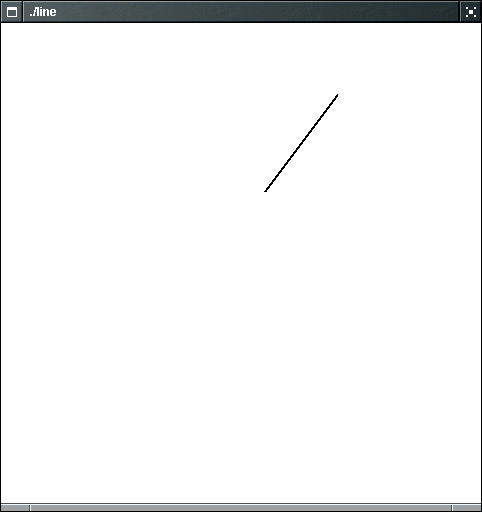## Graphics Structures (Messages)

• You can display text anywhere you like using Message objects.
• You point parameter specifies the upper left corner of the message.
`Point p(1, 3);Message greeting(p, "Hello, Window!");cwin << greeting;`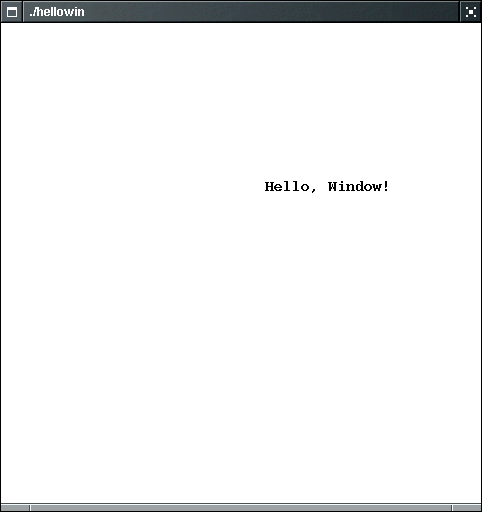## Graphics Structures (move())

• All graphical classes (points, circles, lines, messages) implement the move() member function.
• obj.move(dx, dy) changes the position of the object, moving the entire object by dx units in the x-direction and dy units in the y-direction.
• Either or both of dx and dy can be zero or negative.

## Graphics Structures (square.cpp (Output))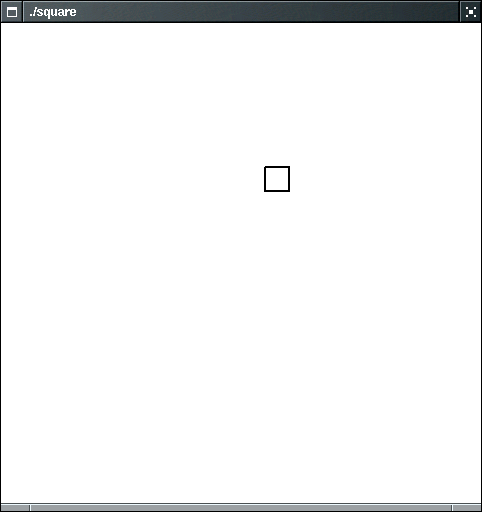## Graphics Structures (Summary)

 Name Purpose Point(x, y) Constructs a point at location (x, y) p.get_x() Returns the x-coordinate of a point p p.get_y() Returns the y-coordinate of a point p p.move(dx, dy) Moves point by by (dx, dy)
 Name Purpose Circle(p, r) Constructs a circle with center p and radius r c.get_center() Returns the center point of a circle c c.get_radius() Returns the radius of a circle c. c.move(dx, dy) Moves circle c by (dx, dy)
 Name Purpose Line(p, q) Constructs a line joining points p and q l.get_start() Returns the starting point of line l l.get_end() Returns the end point of line l l.move(dx, dy) Moves line l by (dx, dy)
 Name Purpose Message(p, s) Constructs a message with starting point p and text string s Message(p, x) Constructs a message with starting point p and label equal to the number x m.get_start() Returns the starting point of message m. m.get_text() Gets the text string message m m.move(dx,dy) Moves message m by (dx, dy)

## Choosing a Coordinate System

• The default coordinate system for the book's graphics classes:
• The origin (0, 0) is at the center.
• x- and y- axis range from -10.0 to 10.0
• The user can reset the coordinate system:
`cwin.coord(x_left, y_top, x_right, y_bottom)`
• Example:

For the following data, the x-axis should range from 1 to 12, and the y-axis should range from 11 to 33:

cwin.coord(1, 11, 12, 33);

 Month Average Temperature Month Average Temperature January 11 July 33 February 13 August 32 March 16 September 29 April 20 October 23 May 25 November 16 June 31 December 12

Output is now in terms of the new coordinate system:

`cwin << Point(1, 11);cwin << Point(2, 13);/* etc. */`

## Getting Input from the Graphics Window

• You cannot use cin to get input from a graphics window.
• To read text input from a graphics window, use the get_string() method.
`string response = cwin.get_string(prompt);`
Example:
`string name = cwin.get_string("Please type your name:");`
• The prompt and input occurs in an input area at either the top or bottom of the graphics window (depending on your system).
• To read numerical data input from a graphics window, use the get_int() or get_double() method.
`int age = cwin.get_int("Please enter your age:");`
• To prompt the user for a mouse input, use the get_mouse() method.
`Point center = cwin.get_mouse("Enter center of circle:");`

## Getting Input from the Graphics Window (click.cpp)

 Name Purpose cwin << x Display the object x (a point, circle, line, message) cwin.cord(x1, y1, x2, y2) Set the coordinate system. (x1, y1) is the top left corner, (x2, y2) is the bottom right corner cwin.get_string(p) Displays prompt p and returns the entered string. cwin.get_int(p) Displays prompt p and returns the entered integer. cwin.get_double(p) Displays prompt p and returns the entered floating-point value. cwin.get_mouse(p) Displays prompt p and returns the mouse click point. cwin.clear() Clears window.

## Comparing Visual and Numerical Information

• Look at the same problem both visually and numerically.
• Determine the intersection between a line and a circle (centered on the screen) - the user specifies a radius and a distance between the horizontal line and the x-axes.
• Plot the line and the circle.
• We would like to know the exact coordinates of the intersection points.
• Calculate the coordinates
• Plot both points and label them with numerical values.
• If you do it right, these two points will show up right on top of the actual intersections in the picture.

## Comparing Visual and Numerical Information (intsect2.cpp)

• If line doesn't meet the circle, then the program will attempt to compute a square root of a negative number, and it will terminate with "Run-time error - math error".
• The protection against this situation will be the topic of the next lecture.